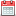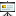# Multi-Time Formalism in Quantum Field Theory44th LQP Workshop "Foundations and Constructive Aspects of QFT"Sascha Lill on October 26, 2019Lill_44_LQP.pdf

In order to describe a Quantum-N-body system in the Schrödinger picture in a manifestly covariant way, one needs to introduce a separate time coordinate $t_k$ for each of the particles $k \in \{1,...,N\}$. The wave function therefore depends on N time coordinates (Multi-Time wave function'') and the Schrödinger equation becomes a system of N Partial Differential Equations (PDEs). Multi-Time wave functions were already introduced in the 1930's and have become a topic of active research again throughout the past years.\\

So far, a series of papers has been published, which investigate the existence and uniqueness of solutions to the Multi-Time Schrödinger equation systems appearing in various quantum mechanical models. However, most of the considerations in these papers were done on a non-rigorous level, for example by checking a certain consistency condition. In my master thesis, I could establish a rigorous proof of existence and uniqueness of a solution to the equations of motion for a Quantum Field Theory (QFT) toy model. This talk will concern about Multi-Time formalism in general, how to construct a solution to the equations of motion and how to show that this PDE system is actually solved.\\